# 图的定义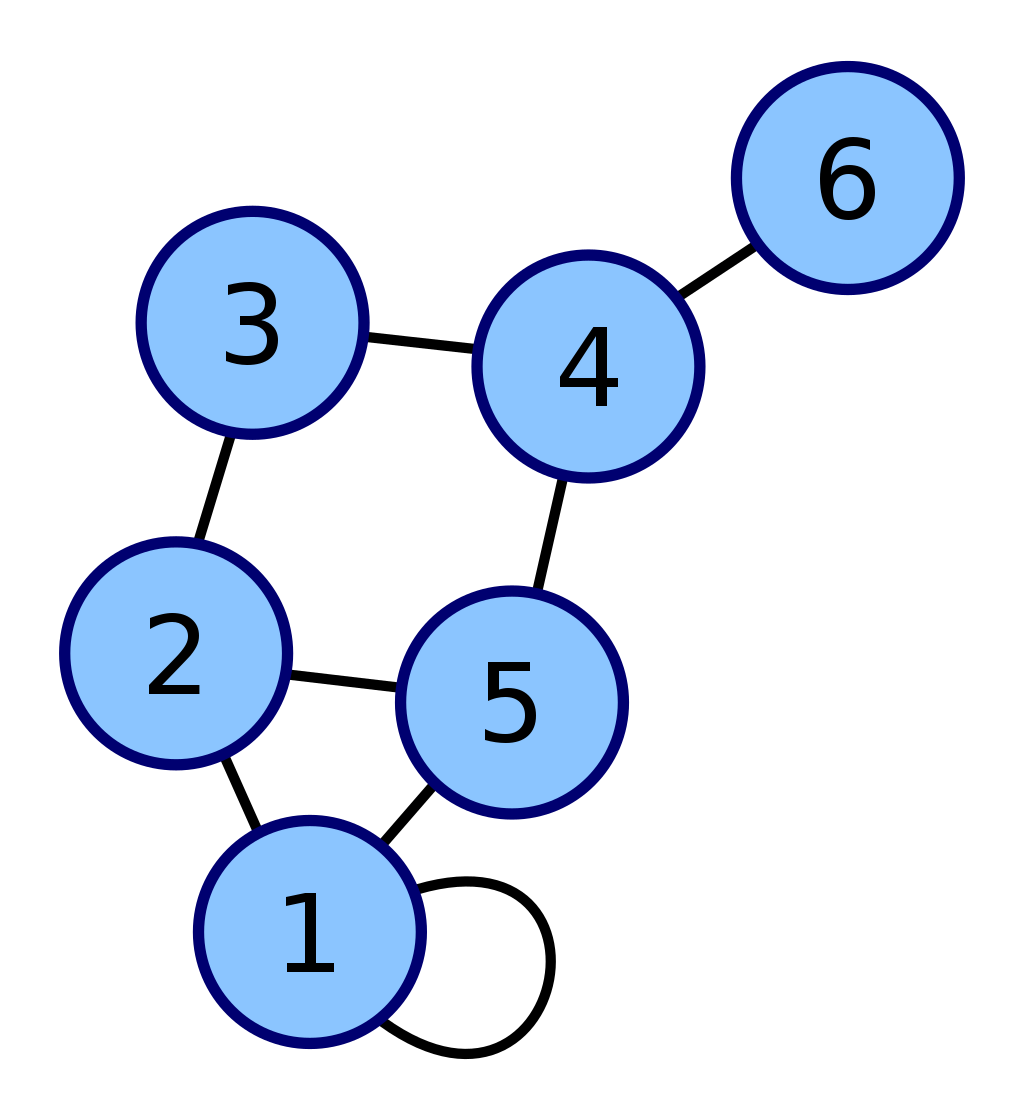（顶点1有一个环的图结构）

• 通常表示为G（V，E）
• G 是一个图
• V 是图 G 中顶点的集合
• E 是图 G 中边的集合。

• 每一个圆圈表示图的一个顶点，顶点放在一起就是 V
• 连接顶点的连线称之为图的边，边放在一起就是 E

## 数据元素的称呼

• 线性表中我们把数据称之为元素
• 树中我们把数据称之为结点
• 图中我们把数据称之为顶点（Vertex）

## 没有数据元素

• 线性表可以没有数据元素，称为空表。
• 树中可以没有结点，称为空树。
• 图一般不为空，我们一般说顶点集合 V 要有穷非空。

## 数据元素的关系

• 线性表中的数据元素，一对一，我们说它们有线性关系。
• 树结构中的数据元素，一对多，我们说相邻两层的结点具有层次关系。
• 图结构中的数据元素，多对多，任意两个顶点之间都可能有关系，顶点之间的逻辑关系用边来表示，边集可以为空，即数据元素可以跟任何其他数据元素无关。

# 图的边

## 无向边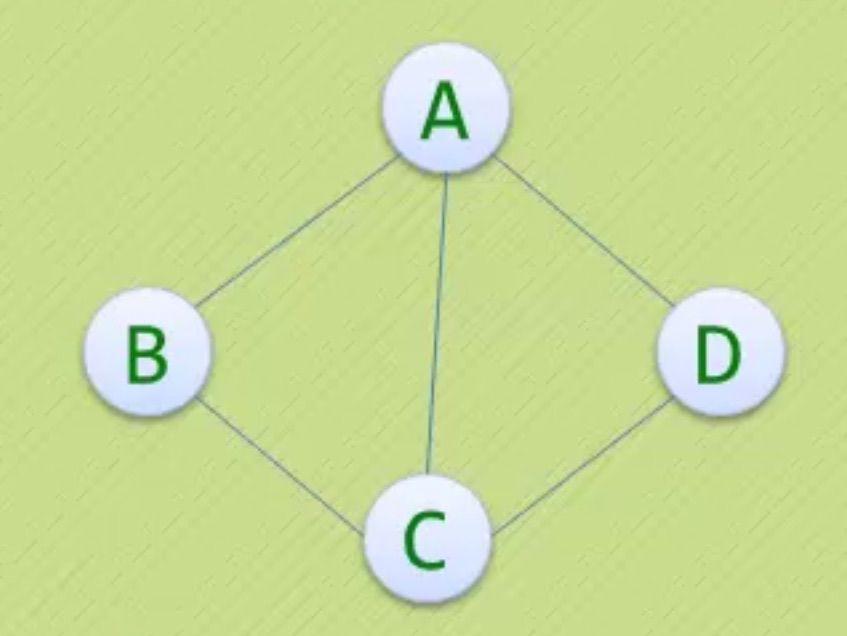• 若顶点 Vi 到 Vj 之间的边没有方向
• 则称这条边为无向边（Edge）
• 用无序偶（Vi，Vj） 来表示
• V 是顶点的集合哈，Vi 和 Vj 就是其中的两个顶点。别忘了。

• 上图G1是一个无向图，如果 $G1=\left { V1 , E2\right }$
• 顶点的集合：$V1=\left{A,B,C,D\right}$
• 边的集合：$E1=\left{(A,B),(B,C),(C,D),(D,A),(A,C)\right}$

## 有向边• 若顶点 Vi 到 Vj 之间的边有方向
• 则称这条边为有向边，也称为弧（Arc）
• 用有序偶 < Vi , Vj > 表示，注意这里是尖括号
• Vi 称为弧尾，Vj 称为弧头。
• 因为是有向边，所有 Vi 和 Vj 顺序不能错，一定是尾巴指向头。

• 上图G2是一个有向图，如果 $G2=\left { V2 , E2\right }$
• 顶点的集合：$V1= \left{ A,B,C,D \right}$
• 边的集合：$E1=\left{(B,A),(B,C),(C,A),(A,D)\right}$
• 这里边的集合要注意顺序！！！

## 简单图

• 若不存在顶点到其自身的边
• 同一条边不重复出现
• 满足以上两个条件的图，叫做简单图。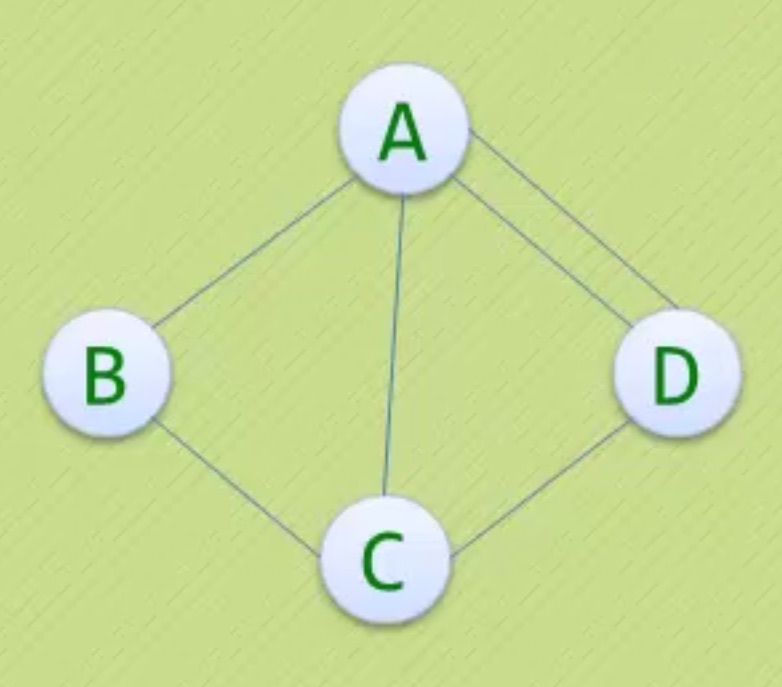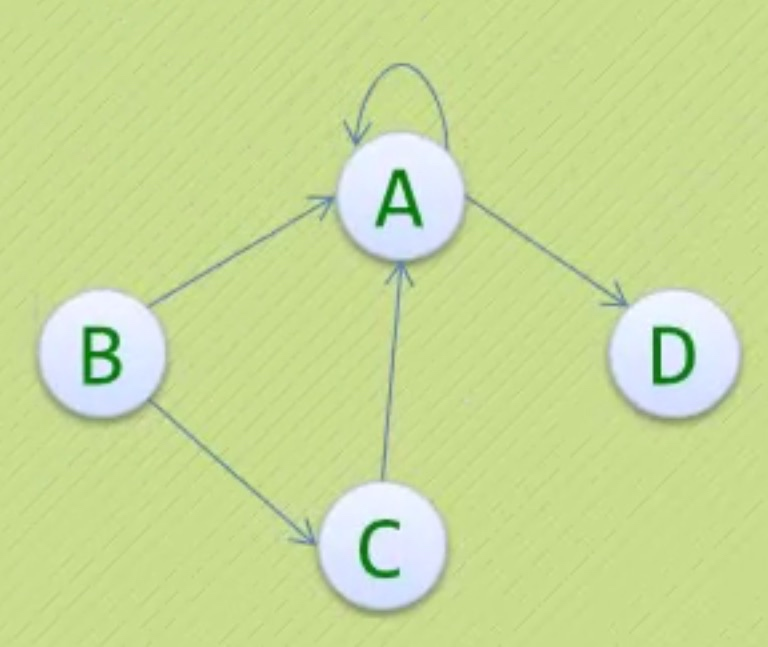# 特殊的图

## 无向完全图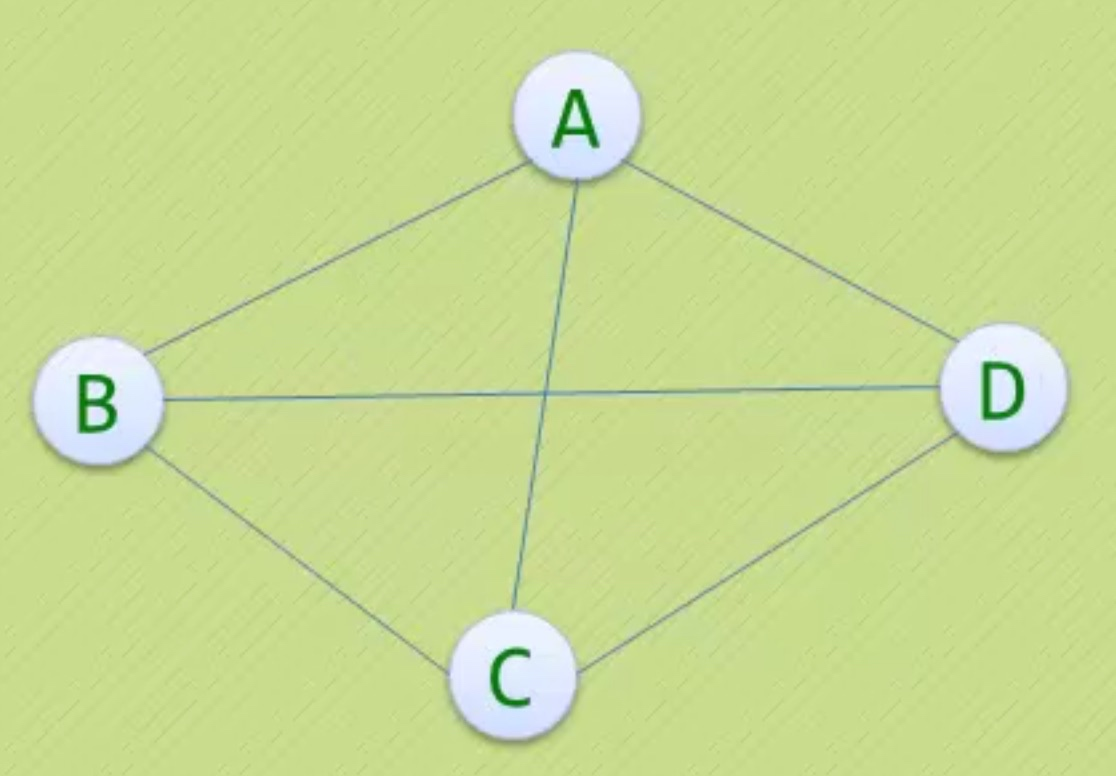• 任意两个顶点之间都存在边
• 则称该图为无向完全图
• n 个顶点的无向完全图有 $n\times(n-1)\div2$ 条边
• 上面就是一张无向完全图，所有顶点都连着边
• ABCD 4个顶点就是 $4\times(4-1)\div2=6$ 条边

## 有向完全图• 任意两个顶点之间都存在方向互为相反的两条弧
• 则称该图为有向完全图
• n 个顶点的无向完全图有 $n\times(n-1)$ 条边
• 上面就是一张无向完全图，所有顶点都与另一个顶点互为相反弧
• ABCD 4个顶点就是 $4\times(4-1)=12$ \条边

## 稀疏图和稠密图

• 边或者弧数小于 $n\times \log n$时的图为稀疏图
• 反之为稠密图

## 带权图

• 图的边或者弧带有与它相关的数字
• 我们把这些相关树称作权（weight）
• 我们称这种带权图为网（NetWork）

## 子图

• 如果 $V2 \subseteq V1$，G2的顶点都在 G1 的顶点的集合内
• 并且！！！
• $E2 \subseteq E1$，G2的边都在 G1 的边的集合内
• 记住，上面两个条件如果同时成立！
• 我么你就说 G2 是 G1 的子图
• 简单就是，我的顶点你也有，我的边你也有，那我就是你的子图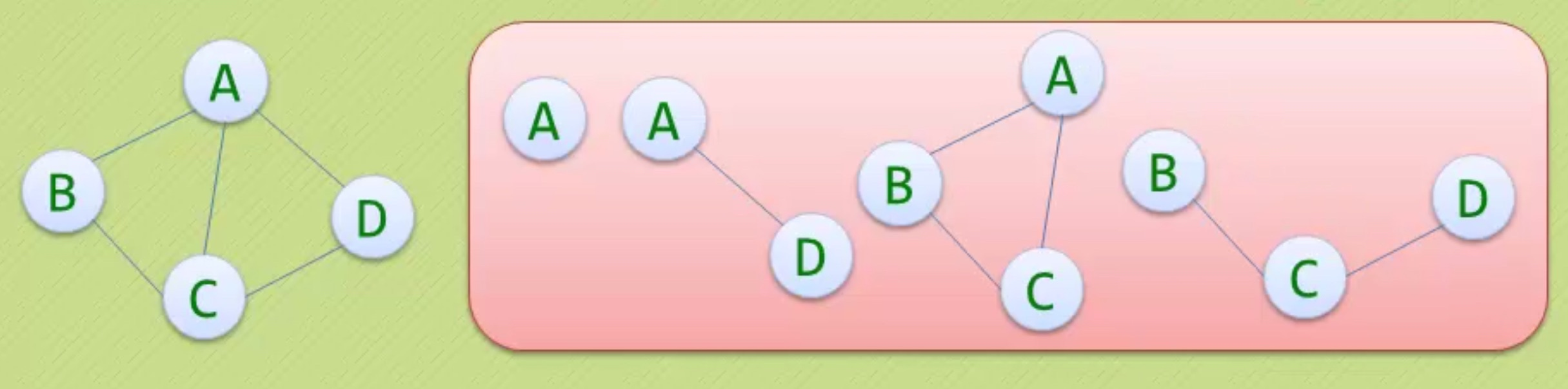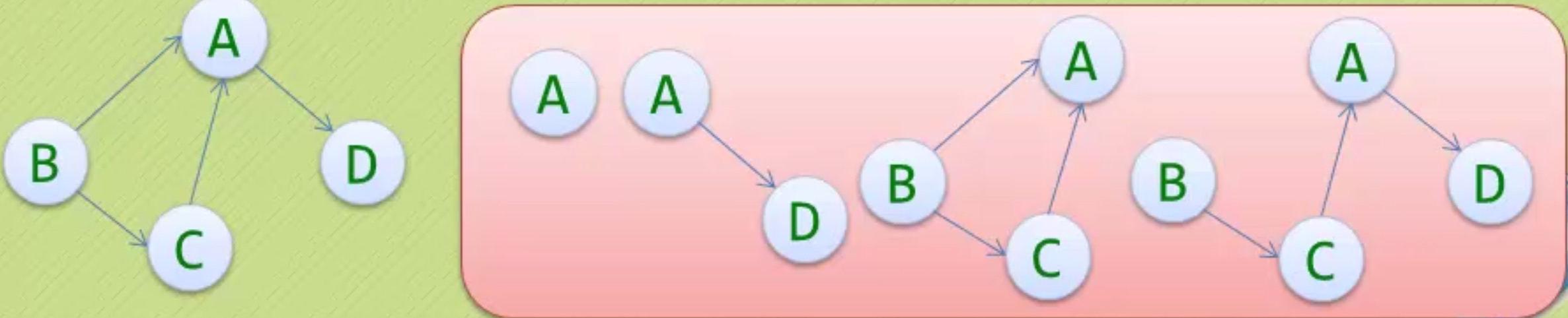# 尾巴

-------------The End-------------